JMSLTM Numerical Library 7.2.0
com.imsl.stat

## Class LinearRegression

• All Implemented Interfaces:
Serializable, Cloneable

```public class LinearRegression
extends Object
implements Serializable, Cloneable```
Fits a multiple linear regression model with or without an intercept. If the constructor argument `hasIntercept` is true, the multiple linear regression model is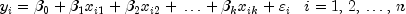where the observed values of the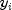's constitute the responses or values of the dependent variable, the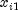's,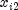's,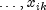's are the settings of the independent variables,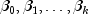are the regression coefficients, and the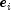's are independently distributed normal errors each with mean zero and variance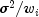. If `hasIntercept` is ``` false```,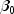is not included in the model.

`LinearRegression` computes estimates of the regression coefficients by minimizing the sum of squares of the deviations of the observed responsefrom the fitted response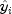for the observations. This minimum sum of squares (the error sum of squares) is in the ANOVA output and denoted by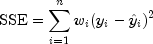In addition, the total sum of squares is output in the ANOVA table. For the case, `hasIntercept` is true; the total sum of squares is the sum of squares of the deviations offrom its mean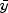--the so-called corrected total sum of squares; it is denoted by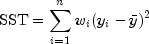For the case `hasIntercept` is `false`, the total sum of squares is the sum of squares of--the so-called uncorrected total sum of squares; it is denoted by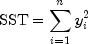See Draper and Smith (1981) for a good general treatment of the multiple linear regression model, its analysis, and many examples.

In order to compute a least-squares solution, ```LinearRegression ``` performs an orthogonal reduction of the matrix of regressors to upper triangular form. Givens rotations are used to reduce the matrix. This method has the advantage that the loss of accuracy resulting from forming the crossproduct matrix used in the normal equations is avoided, while not requiring the storage of the full matrix of regressors. The method is described by Lawson and Hanson, pages 207-212.

From a general linear model fitted using the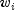's as the weights, inner class `LinearRegression.CaseStatistics` can also compute predicted values, confidence intervals, and diagnostics for detecting outliers and cases that greatly influence the fitted regression. Let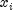be a column vector containing elements of the-th row of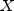. Let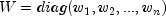. The leverage is defined as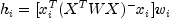(In the case of linear equality restrictions on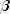, the leverage is defined in terms of the reduced model.) Put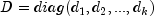with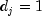if the-th diagonal element of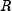is positive and 0 otherwise. The leverage is computed as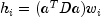whereis a solution to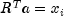. The estimated variance of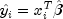is given by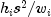, where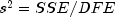. The computation of the remainder of the case statistics follows easily from their definitions.

Letdenote the residual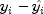for theth case. The estimated variance ofis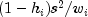where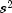is the residual mean square from the fitted regression. Theth standardized residual (also called the internally studentized residual) is by definition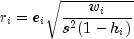and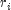follows an approximate standard normal distribution in large samples.

Theth jackknife residual or deleted residual involves the difference betweenand its predicted value based on the data set in which theth case is deleted. This difference equals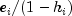. The jackknife residual is obtained by standardizing this difference. The residual mean square for the regression in which theth case is deleted is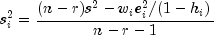The jackknife residual is defined to be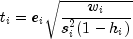and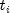follows adistribution with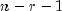degrees of freedom.

Cook's distance for theth case is a measure of how much an individual case affects the estimated regression coefficients. It is given by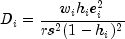Weisberg (1985) states that if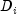exceeds the 50-th percentile of the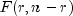distribution, it should be considered large. (This value is about 1. This statistic does not have an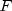distribution.)

DFFITS, like Cook's distance, is also a measure of influence. For theth case, DFFITS is computed by the formula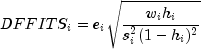Hoaglin and Welsch (1978) suggest that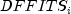greater than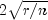is large.

Often predicted values and confidence intervals are desired for combinations of settings of the effect variables not used in computing the regression fit. This can be accomplished using a single data matrix by including these settings of the variables as part of the data matrix and by setting the response equal to `Double.NaN`. ``` LinearRegression``` will omit the case when performing the fit and a predicted value and confidence interval for the missing response will be computed from the given settings of the effect variables.

Example1, Example2, Serialized Form
• ### Nested Class Summary

Nested Classes
Modifier and Type Class and Description
`class ` `LinearRegression.CaseStatistics`
Inner Class `CaseStatistics` allows for the computation of predicted values, confidence intervals, and diagnostics for detecting outliers and cases that greatly influence the fitted regression.
`class ` `LinearRegression.CoefficientTTests`
Contains statistics related to the regression coefficients.
• ### Constructor Summary

Constructors
Constructor and Description
```LinearRegression(int nVariables, boolean hasIntercept)```
Constructs a new linear regression object.
• ### Method Summary

Methods
Modifier and Type Method and Description
`ANOVA` `getANOVA()`
Get an analysis of variance table and related statistics.
`LinearRegression.CaseStatistics` ```getCaseStatistics(double[] x, double y)```
Returns the case statistics for an observation.
`LinearRegression.CaseStatistics` ```getCaseStatistics(double[] x, double y, double w)```
Returns the case statistics for an observation and a weight.
`LinearRegression.CaseStatistics` ```getCaseStatistics(double[] x, double y, double w, int pred)```
Returns the case statistics for an observation, weight, and future response count for the desired prediction interval.
`LinearRegression.CaseStatistics` ```getCaseStatistics(double[] x, double y, int pred)```
Returns the case statistics for an observation and future response count for the desired prediction interval.
`double[]` `getCoefficients()`
Returns the regression coefficients.
`LinearRegression.CoefficientTTests` `getCoefficientTTests()`
Returns statistics relating to the regression coefficients.
`double[][]` `getR()`
Returns a copy of the R matrix.
`int` `getRank()`
Returns the rank of the matrix.
`void` ```update(double[][] x, double[] y)```
Updates the regression object with a new set of observations.
`void` ```update(double[][] x, double[] y, double[] w)```
Updates the regression object with a new set of observations and weights.
`void` ```update(double[] x, double y)```
Updates the regression object with a new observation.
`void` ```update(double[] x, double y, double w)```
Updates the regression object with a new observation and weight.
• ### Methods inherited from class java.lang.Object

`clone, equals, finalize, getClass, hashCode, notify, notifyAll, toString, wait, wait, wait`
• ### Constructor Detail

• #### LinearRegression

```public LinearRegression(int nVariables,
boolean hasIntercept)```
Constructs a new linear regression object.
Parameters:
`nVariables` - `int` number of variables in the regression
`hasIntercept` - `boolean` which indicates whether or not an intercept is in this regression model
• ### Method Detail

• #### getANOVA

`public ANOVA getANOVA()`
Get an analysis of variance table and related statistics.
Returns:
an `ANOVA` table and related statistics
• #### getCaseStatistics

```public LinearRegression.CaseStatistics getCaseStatistics(double[] x,
double y)```
Returns the case statistics for an observation.
Parameters:
`x` - a `double` array containing the independent (explanatory) variables. Its length must be equal to the number of variables set in the LinearRegression constructor.
`y` - a `double` representing the dependent (response) variable
Returns:
the CaseStatistics for the observation.
• #### getCaseStatistics

```public LinearRegression.CaseStatistics getCaseStatistics(double[] x,
double y,
double w)```
Returns the case statistics for an observation and a weight.
Parameters:
`x` - a `double` array containing the independent (explanatory) variables. Its length must be equal to the number of variables set in the constructor.
`y` - a `double` representing the dependent (response) variable
`w` - a `double` representing the weight
Returns:
the CaseStatistics for the observation.
• #### getCaseStatistics

```public LinearRegression.CaseStatistics getCaseStatistics(double[] x,
double y,
double w,
int pred)```
Returns the case statistics for an observation, weight, and future response count for the desired prediction interval.
Parameters:
`x` - a `double` array containing the independent (explanatory) variables. Its length must be equal to the number of variables set in the constructor.
`y` - a `double` representing the dependent (response) variable
`w` - a `double` representing the weight
`pred` - an `int` representing the number of future responses for which the prediction interval is desired on the average of the future responses
Returns:
the CaseStatistics for the observation.
• #### getCaseStatistics

```public LinearRegression.CaseStatistics getCaseStatistics(double[] x,
double y,
int pred)```
Returns the case statistics for an observation and future response count for the desired prediction interval.
Parameters:
`x` - a `double` array containing the independent (explanatory) variables. Its length must be equal to the number of variables set in the constructor.
`y` - a `double` representing the dependent (response) variable
`pred` - an `int` representing the number of future responses for which the prediction interval is desired on the average of the future responses.
Returns:
the CaseStatistics for the observation.
• #### getCoefficients

`public double[] getCoefficients()`
Returns the regression coefficients.
Returns:
a `double` array containing the regression coefficients. If `hasIntercept` is `false` its length is equal to the number of variables. If ``` hasIntercept``` is `true` then its length is the number of variables plus one and the 0-th entry is the value of the intercept. If the model is not full rank, the regression coefficients are not uniquely determined. In this case, a warning is issued and a solution with all linearly dependent regressors set to zero is returned.
`Warning`
• #### getCoefficientTTests

`public LinearRegression.CoefficientTTests getCoefficientTTests()`
Returns statistics relating to the regression coefficients.
• #### getR

`public double[][] getR()`
Returns a copy of the R matrix. R is the upper triangular matrix containing the R matrix from a QR decomposition of the matrix of regressors.
Returns:
a `double` matrix containing a copy of the R matrix
• #### getRank

`public int getRank()`
Returns the rank of the matrix.
Returns:
the `int` rank of the matrix
• #### update

```public void update(double[][] x,
double[] y)```
Updates the regression object with a new set of observations.
Parameters:
`x` - a `double` matrix containing the independent (explanatory) variables. The number of rows in `x` must equal the length of `y` and the number of columns must be equal to the number of variables set in the constructor.
`y` - a `double` array containing the dependent (response) variables.
• #### update

```public void update(double[][] x,
double[] y,
double[] w)```
Updates the regression object with a new set of observations and weights.
Parameters:
`x` - a `double` matrix containing the independent (explanatory) variables. The number of rows in `x` must equal the length of `y` and the number of columns must be equal to the number of variables set in the constructor.
`y` - a `double` array containing the dependent (response) variables.
`w` - a `double` array representing the weights
• #### update

```public void update(double[] x,
double y)```
Updates the regression object with a new observation.
Parameters:
`x` - a `double` array containing the independent (explanatory) variables. Its length must be equal to the number of variables set in the constructor.
`y` - a `double` representing the dependent (response) variable
• #### update

```public void update(double[] x,
double y,
double w)```
Updates the regression object with a new observation and weight.
Parameters:
`x` - a `double` array containing the independent (explanatory) variables. Its length must be equal to the number of variables set in the constructor.
`y` - a `double` representing the dependent (response) variable
`w` - a `double` representing the weight
JMSLTM Numerical Library 7.2.0